# GMAT Math : Calculating the height of a right triangle

## Example Questions

### Example Question #1 : Calculating The Height Of A Right Triangle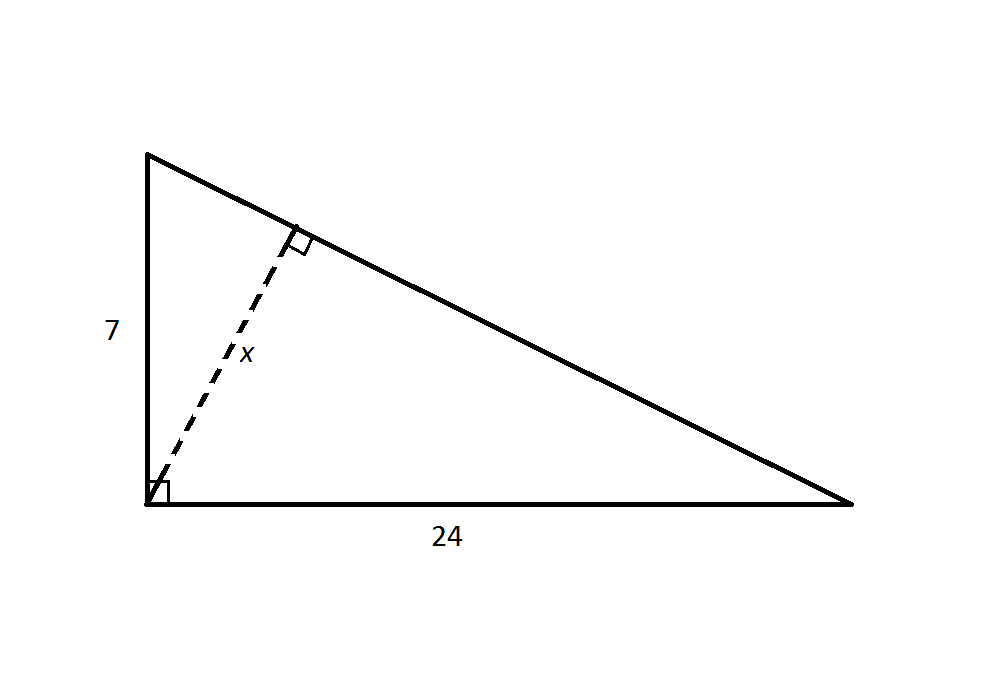Note: Figure NOT drawn to scale

Refer to the above diagram.

Calculate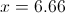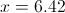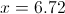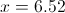Insufficient information is given to calculate.Explanation:

The hypotenuse of the large right triangle isThe area of the large right triangle is half the product of its base and its height. The base can be any side of the triangle; the height would be the length of the altitude, which is the perpendicular segment from the opposite vertex to that base.

Therefore, the area of the triangle can be calculated as half the product of the legs: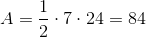Or half the product of the hypotenuse and the lengthof the dashed line.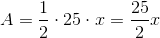To calculate, we can set these expressions equal to each other: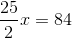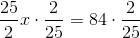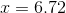### Example Question #2 : Calculating The Height Of A Right Triangle

A right triangle has a base of 8 and an area of 24. What is the height of the triangle?Explanation:

Using the formula for the area of a right triangle, we can plug in the given values and solve for the height of the triangle: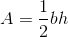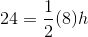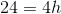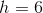### Example Question #3 : Calculating The Height Of A Right Triangle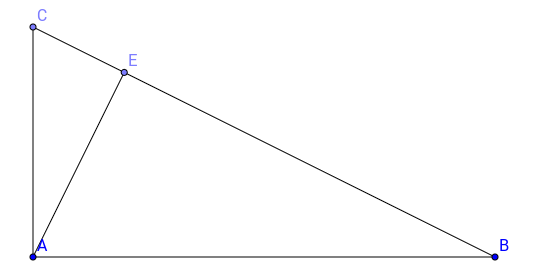Triangle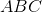is a right triangle with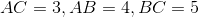. What is the length of its height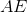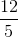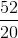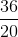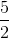Explanation:

The height AE, divides the triangle ABC, in two triangles AEC and AEB with same proportions as the original triangle ABC, this property holds true for any right triangle.

In other words,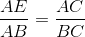.

Therefore, we can calculate, the length of AE: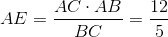.

### Example Question #4 : Calculating The Height Of A Right TriangleTriangleis a right triangle with sides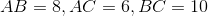. What is the size of the height?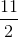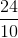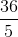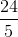Explanation:

As we have previously seen, the height of a right triangle divides a it into two similar triangles with sides of same proportion.

Therefore, we can set up the following equality:or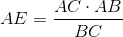.

By plugging in the numbers, we get,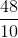or.

### Example Question #5 : Calculating The Height Of A Right Triangle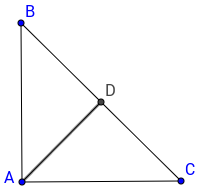is a right isosceles triangle, with height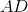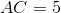, what is the length of the height?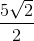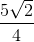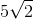Explanation:

Since here ABC is a isosceles right triangle, its height is half the size of the hypotenuse.

We just need to apply the Pythagorean Theorem to get the length of BC, and divide this length by two.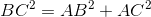, so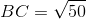.

Therefore,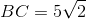and the final answer is.

Tired of practice problems?

Try live online GMAT Math prep today.